# Electrochemistry Part I Redox Review How to Balance

• Slides: 14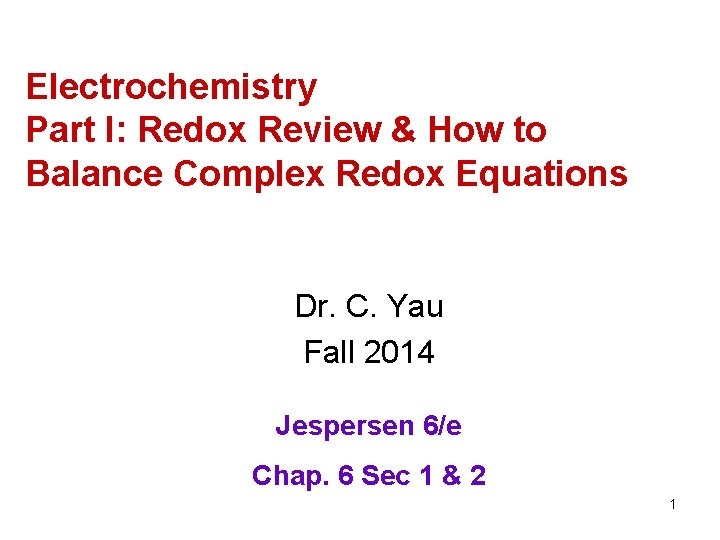Electrochemistry Part I: Redox Review & How to Balance Complex Redox Equations Dr. C. Yau Fall 2014 Jespersen 6/e Chap. 6 Sec 1 & 2 1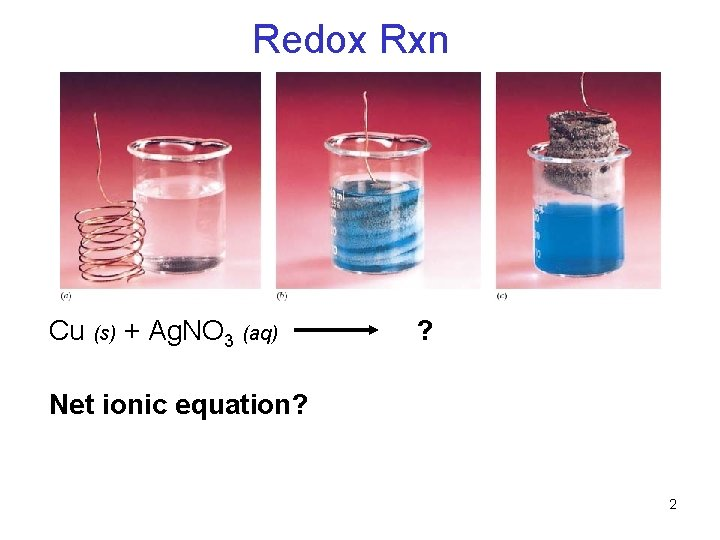Redox Rxn Cu (s) + Ag. NO 3 (aq) ? Net ionic equation? 2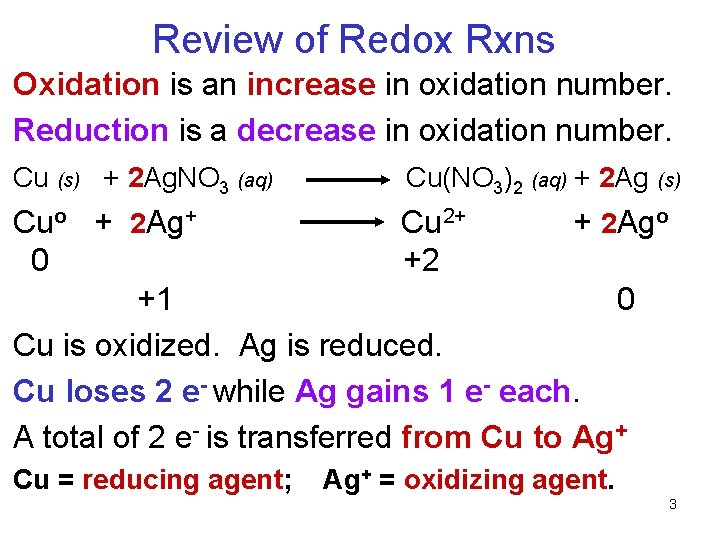Review of Redox Rxns Oxidation is an increase in oxidation number. Reduction is a decrease in oxidation number. Cu (s) + 2 Ag. NO 3 (aq) Cu(NO 3)2 (aq) + 2 Ag (s) Cuo + 2 Ag+ Cu 2+ + 2 Ago 0 +2 +1 0 Cu is oxidized. Ag is reduced. Cu loses 2 e- while Ag gains 1 e- each. A total of 2 e- is transferred from Cu to Ag+ Cu = reducing agent; Ag+ = oxidizing agent. 3Alternatively, you can remember… LEO the lion says GER Loss of Electrons is Oxidation. Gain of Electrons is Reduction. Cu + Ag+ Cu Ag+ + e- Cu 2+ + Ag Cu 2+ + 2 e. Ag Oxidation (Loss of e-) Reduction (Gain of e-) 4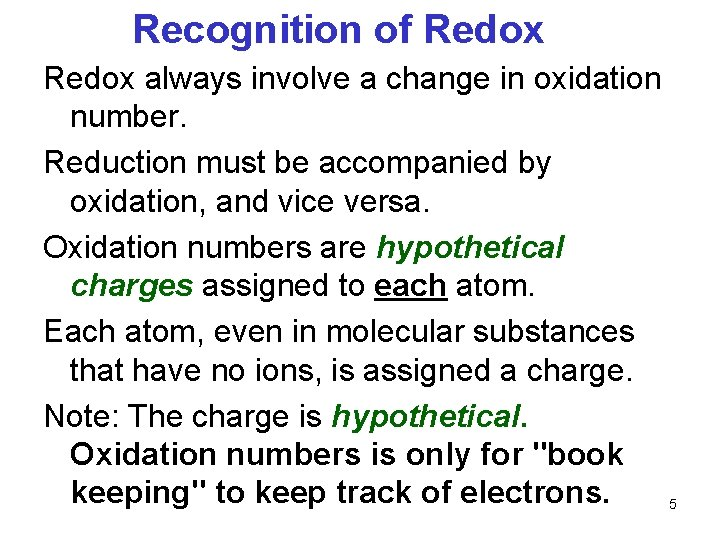Recognition of Redox always involve a change in oxidation number. Reduction must be accompanied by oxidation, and vice versa. Oxidation numbers are hypothetical charges assigned to each atom. Each atom, even in molecular substances that have no ions, is assigned a charge. Note: The charge is hypothetical. Oxidation numbers is only for "book keeping" to keep track of electrons. 5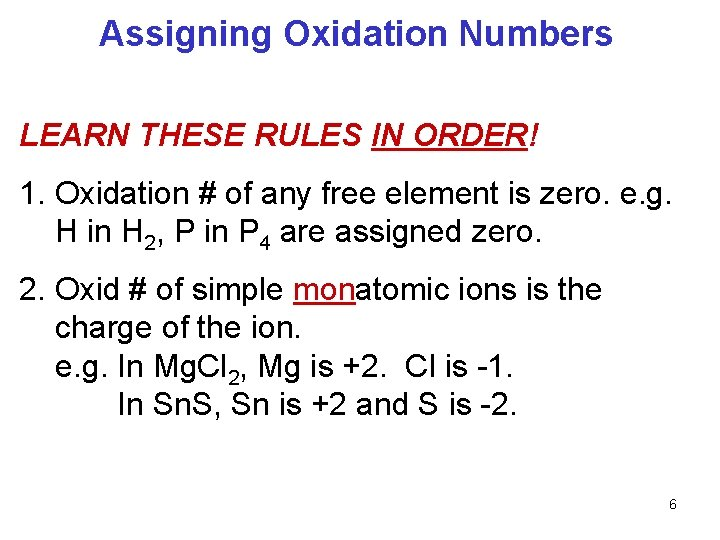Assigning Oxidation Numbers LEARN THESE RULES IN ORDER! 1. Oxidation # of any free element is zero. e. g. H in H 2, P in P 4 are assigned zero. 2. Oxid # of simple monatomic ions is the charge of the ion. e. g. In Mg. Cl 2, Mg is +2. Cl is -1. In Sn. S, Sn is +2 and S is -2. 6Assigning Oxidation Numbers 3. In its cmpd, F is assigned -1. 4. In its cmpds, H is +1 unless it is bonded to a metal where it is -1. e. g. In HCl, H is +1 and Cl is -1. In Mg. H 2, Mg is +2 and H is -1. 5. In its cmpds, O is -2 unless it is a peroxide. eg. in Mg. O, Mg is +2, O is -2. In hydrogen peroxide (H 2 O 2), H is +1 and O is -1. 7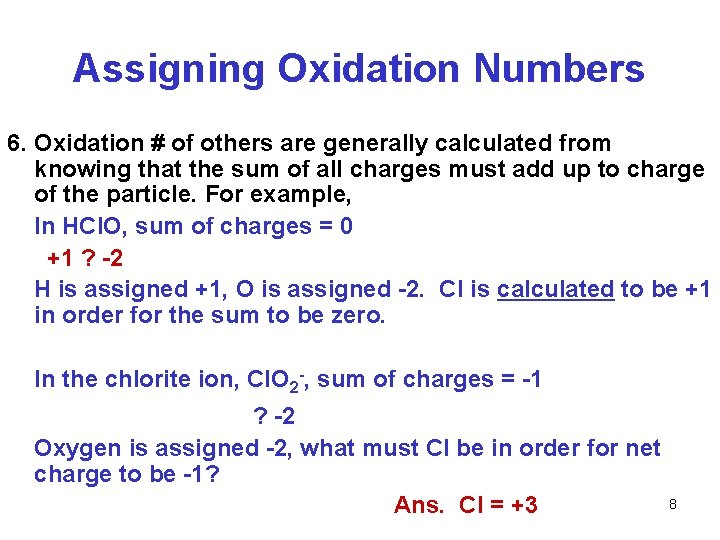Assigning Oxidation Numbers 6. Oxidation # of others are generally calculated from knowing that the sum of all charges must add up to charge of the particle. For example, In HCl. O, sum of charges = 0 +1 ? -2 H is assigned +1, O is assigned -2. Cl is calculated to be +1 in order for the sum to be zero. In the chlorite ion, Cl. O 2 -, sum of charges = -1 ? -2 Oxygen is assigned -2, what must Cl be in order for net charge to be -1? Ans. Cl = +3 8Give the oxidation number: 1. N in NO 3 - 2. Cr in Cr. SO 4 3. S in S 2 O 42 Identify which is the oxidizing agent: Cr 2 O 72− + 14 H+ + 6 Cl− 3 Cl 2 + 2 Cr 3+ + 7 H 2 O 9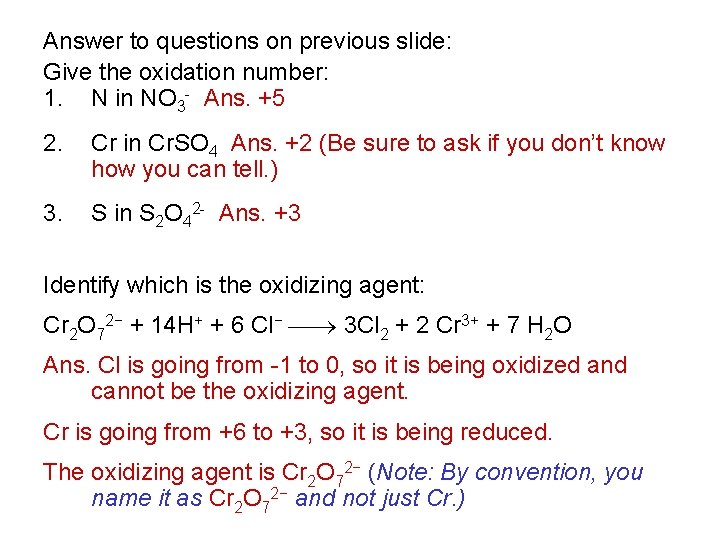Answer to questions on previous slide: Give the oxidation number: 1. N in NO 3 - Ans. +5 2. Cr in Cr. SO 4 Ans. +2 (Be sure to ask if you don’t know how you can tell. ) 3. S in S 2 O 42 - Ans. +3 Identify which is the oxidizing agent: Cr 2 O 72− + 14 H+ + 6 Cl− 3 Cl 2 + 2 Cr 3+ + 7 H 2 O Ans. Cl is going from -1 to 0, so it is being oxidized and cannot be the oxidizing agent. Cr is going from +6 to +3, so it is being reduced. The oxidizing agent is Cr 2 O 72− (Note: By convention, you name it as Cr 2 O 72− and not just Cr. )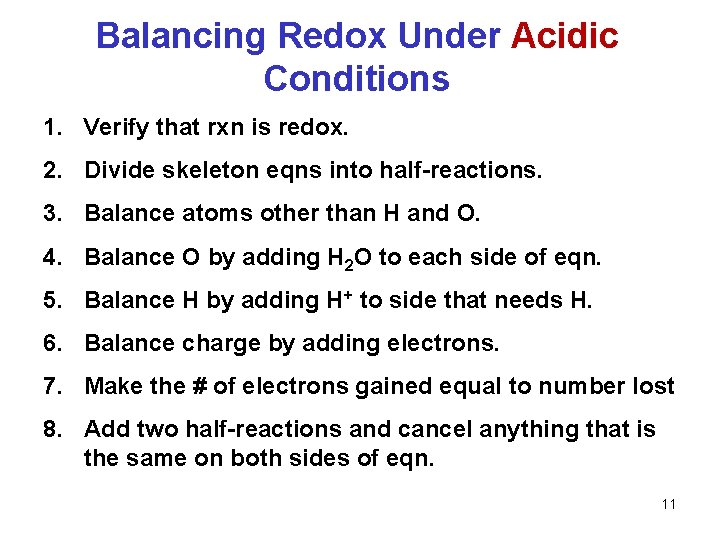Balancing Redox Under Acidic Conditions 1. Verify that rxn is redox. 2. Divide skeleton eqns into half-reactions. 3. Balance atoms other than H and O. 4. Balance O by adding H 2 O to each side of eqn. 5. Balance H by adding H+ to side that needs H. 6. Balance charge by adding electrons. 7. Make the # of electrons gained equal to number lost 8. Add two half-reactions and cancel anything that is the same on both sides of eqn. 11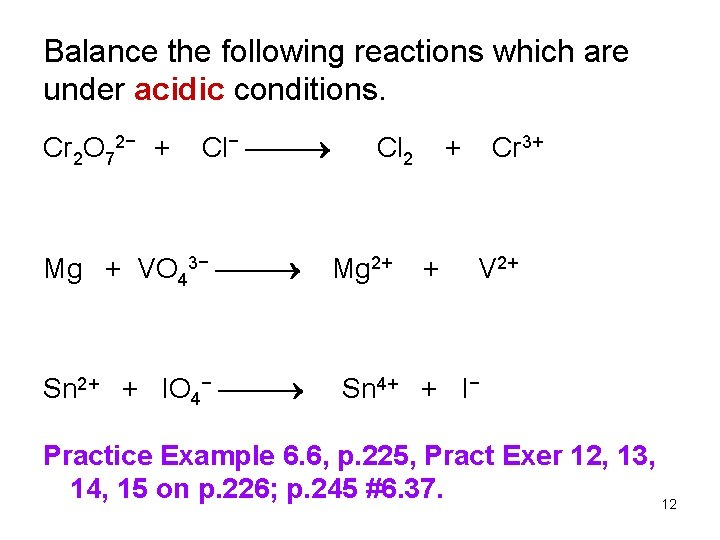Balance the following reactions which are under acidic conditions. Cr 2 O 72− + Cl− Mg + VO 43− Sn 2+ + IO 4− Cl 2 Mg 2+ + + Cr 3+ V 2+ Sn 4+ + I− Practice Example 6. 6, p. 225, Pract Exer 12, 13, 14, 15 on p. 226; p. 245 #6. 37. 12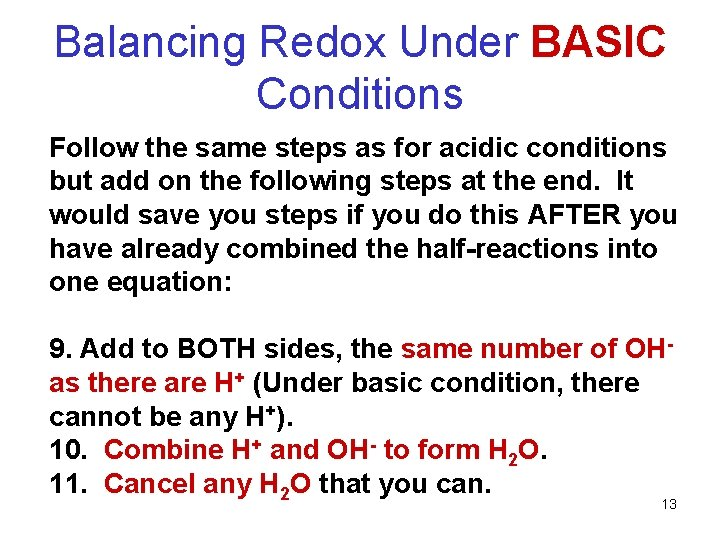Balancing Redox Under BASIC Conditions Follow the same steps as for acidic conditions but add on the following steps at the end. It would save you steps if you do this AFTER you have already combined the half-reactions into one equation: 9. Add to BOTH sides, the same number of OHas there are H+ (Under basic condition, there cannot be any H+). 10. Combine H+ and OH- to form H 2 O. 11. Cancel any H 2 O that you can. 13Balance the following reactions which are under basic conditions. 1. SO 32– + Mn. O 4– SO 42– + Mn. O 2 Which is the reducing agent? 2. CN– + Mn. O 4– SO 32 - CNO– + Mn. O 2 Which is the oxidizing agent? Practice Exer 6. 16, 17, 18, 19 on p. 227 and p. 245 #6. 39 Mn. O 414